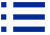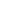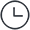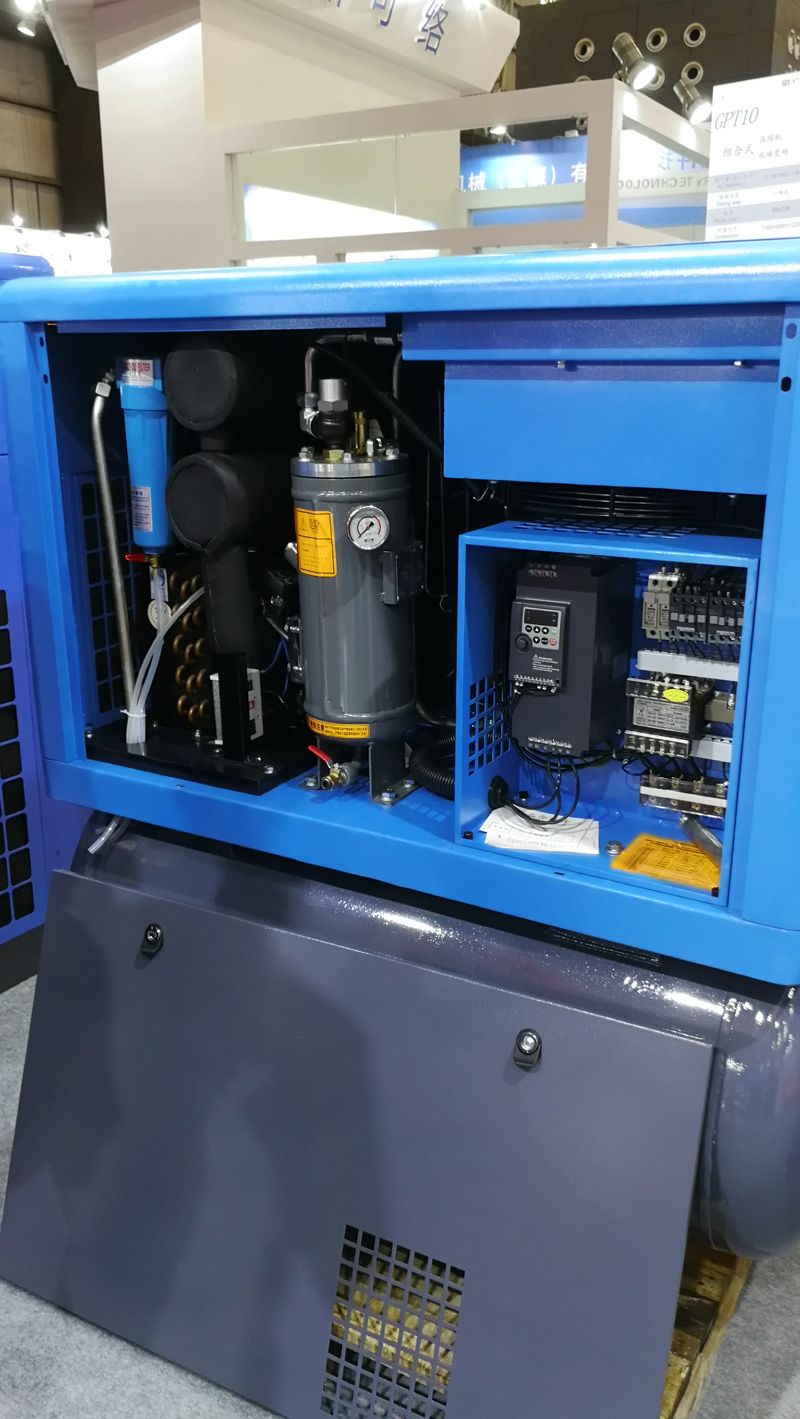About usclassification### S800V-for-air-compressor2020-08-22Number of visits：2249BackS800V used on the air compressor application

Basic function parameter setting:

P0.01=2

P0.10=50

P0.12=50

P1.01= motor rated power

P1.02=motor rated voltage

P1.03= motor rated current

P1.04=motor rated frequency

P1.05= motor rated RPM

P0.02=2

P0.03=9

P0.17=15

P0.18=15

P0.14=25

P5.02=2

Search